﻿ MTH 317:Mathematics (4) | Department of Electronics and Electrical Communications Engineering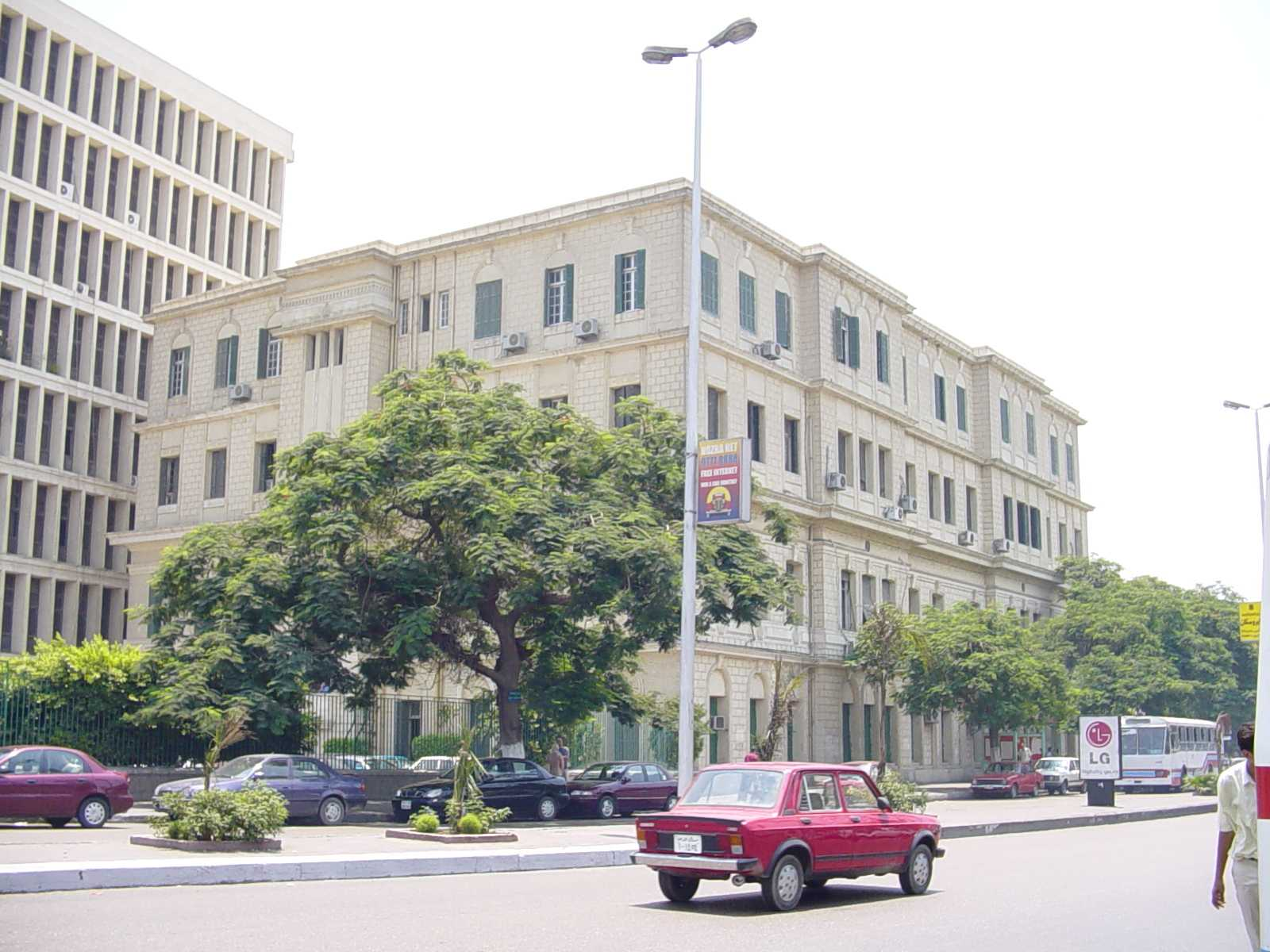## EECE Department Building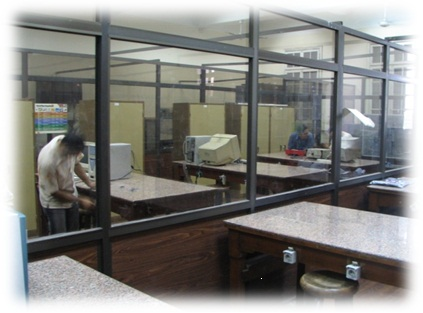## Electronics Lab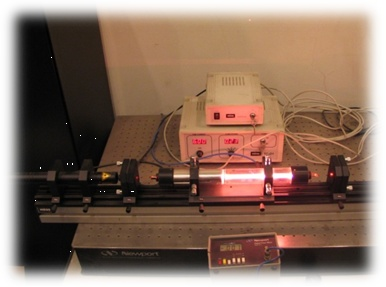## Laser Lab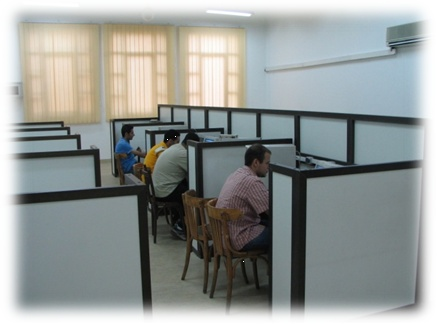## Hammam Lab

Languages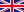User login
Links

## MTH 317:Mathematics (4)

 Academic Year : 3rd Year Academic Term : 1st Term Instructor(s) : Weekly Hours: Lectures : 3 Exercises : 1 Grading System : Term Work : 30 Lab/Oral : Final Exam : 70 Final Exam Hours : 2 Evaluation Link : Selection Link : Web Page Link : Contents : Basic probability theory, Set theory, Conditional probabilities, Random variables, Probability distribution functions, Functions of random variables, Continuous and discrete distribution functions, Special functions: beta and gamma functions, error function, and Bessel function – Partial differential equations and introduction to finite differences method – Discrete mathematics